``` Analysis of the 2000 Florida presidential balloting with program code and graphical output by Roger Luebeck © 2000, 2017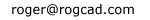---------------- ```
 home Balloting Simple trig Logistic map The Attractor of Henon Number doubling Barnsley's Fern The Sierpinski Triangle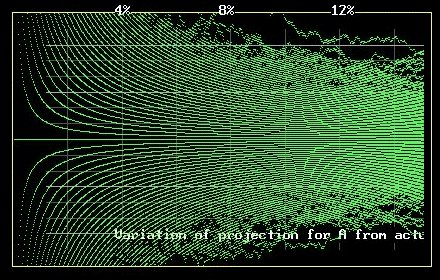``` Output: First 20 percent Amplitude: .5 X increment: .04 Votes: 20000 Trials: 500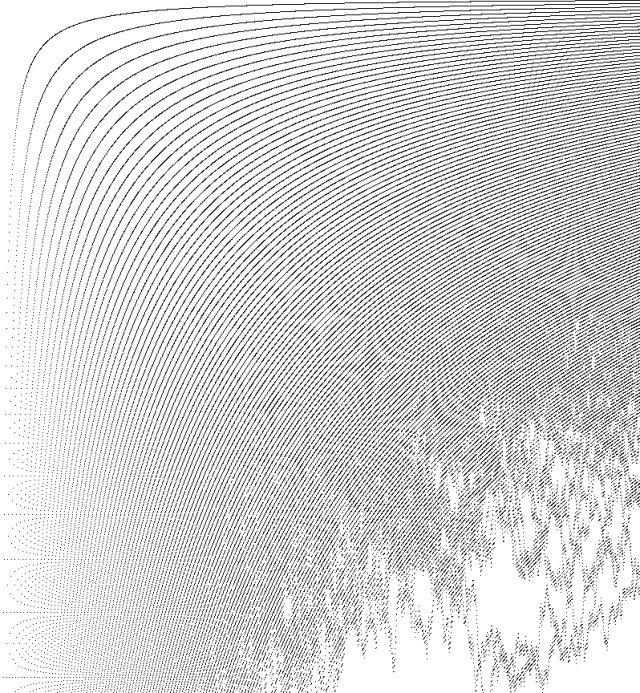Output: First 50 percent Amplitude: .5 X increment: .04 Votes: 20000 Trials: 500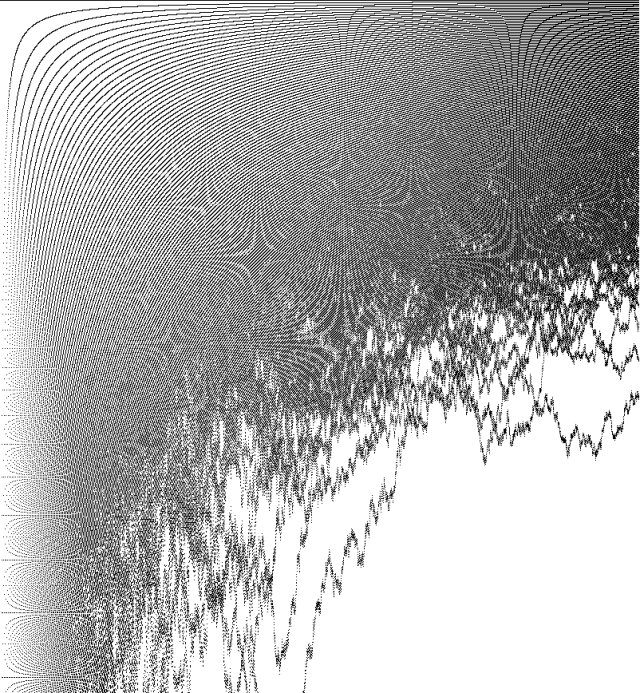Output: First 80 percent Amplitude: .5 X increment: .04 Votes: 20000 Trials: 500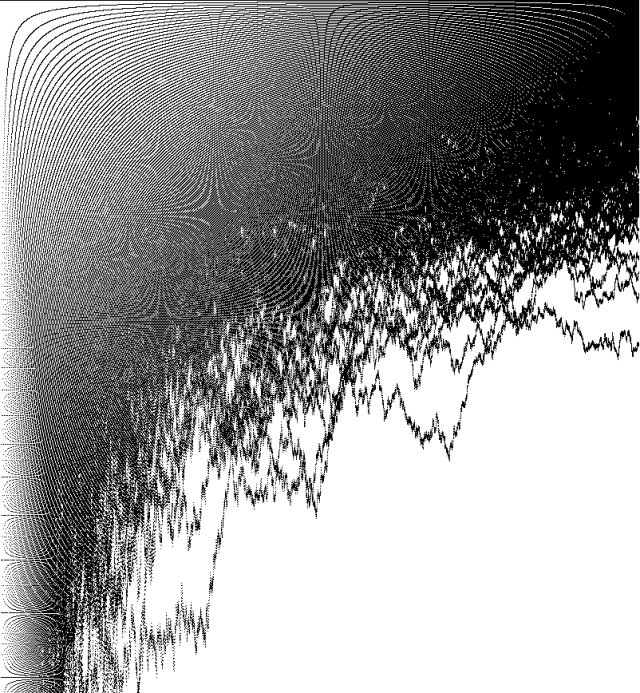More amazement: When I shrunk the images, even more accentuated and previously undetected patterns appeared. The greater the shrinkage, the more revealing are the newly seen patterns. The following three images are 1/2 size, 1/4 size and 1/6 size (then re-enlarged) of the "50 percent" image: Output: First 50 percent Amplitude: .5 X increment: .04 Votes: 20000 Trials: 500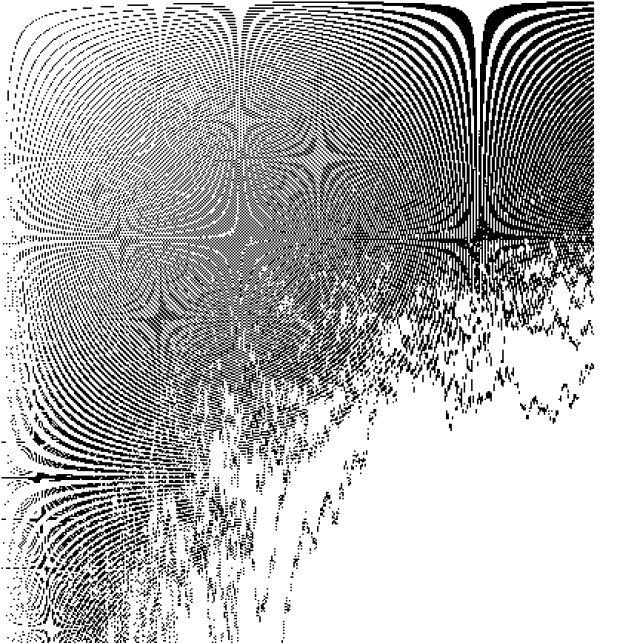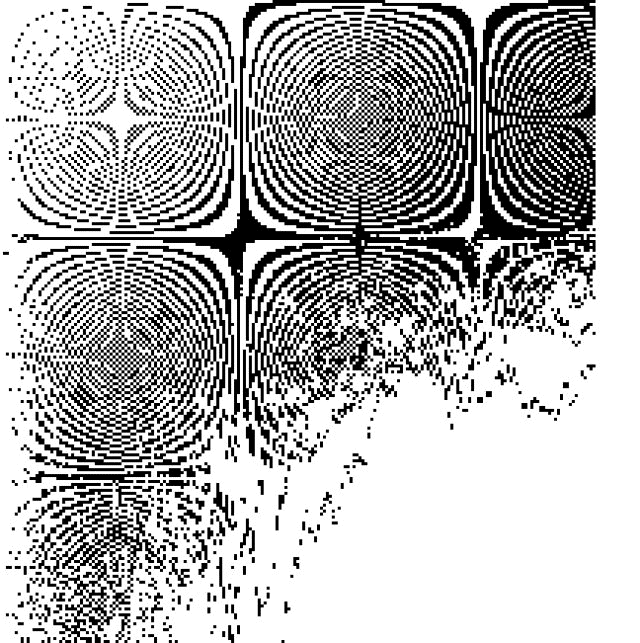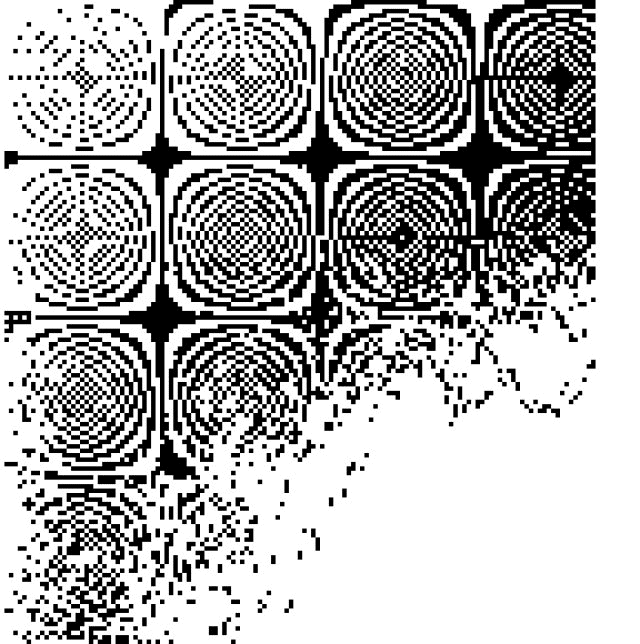Strangely enough, I discovered this identical pattern when I graphed the logistic difference equation in a circular manner for a given value of the parameter r. Those images are displayed on the logistic difference equation page of this website. Just as surprising, this very same pattern showed up on one of my 3D-CAD images, due to some odd combination of perspective angle and the ratio of cross-hatch lines used to color a plane. I've never seen that pattern or any other pattern appearing on any of my thousands of CAD images. Here it is: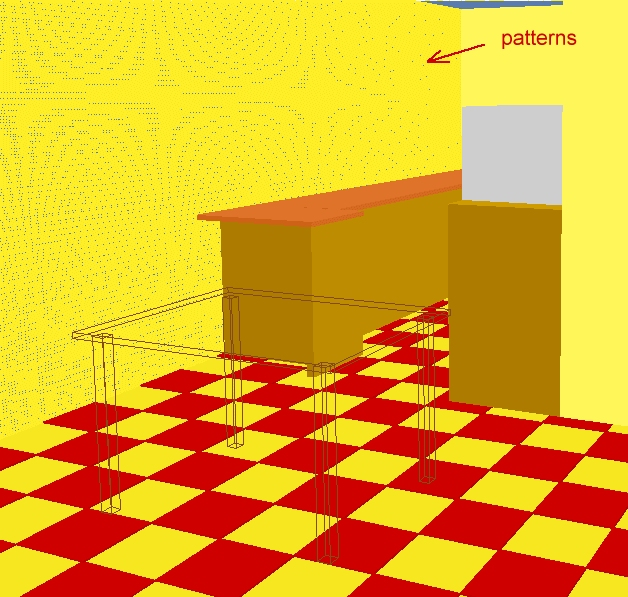Here is an image from my original DOS BASIC program, which I used to determine the margin of error in my prediction: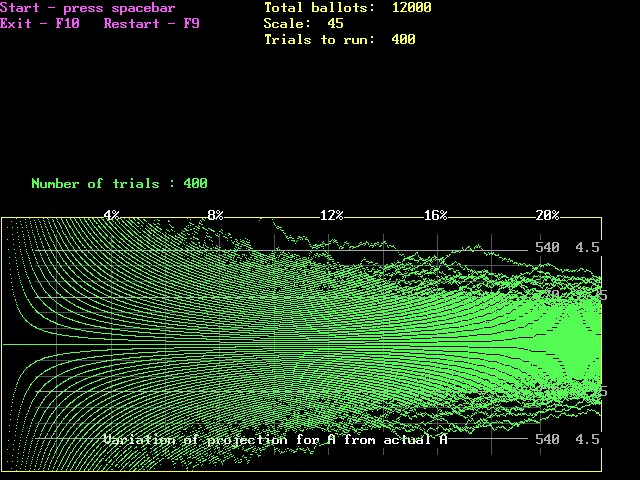By varying the scale and the percent of output, one can adjust the manner in which the patterns appear:Shrinking the above image gradually revealed some grouping of the Y values. See next two images: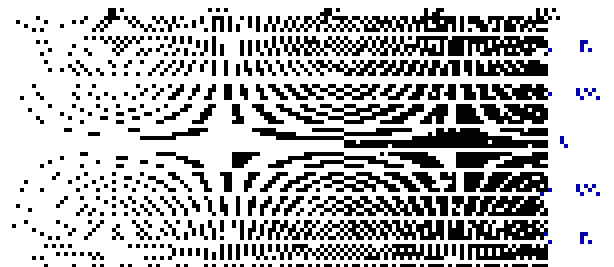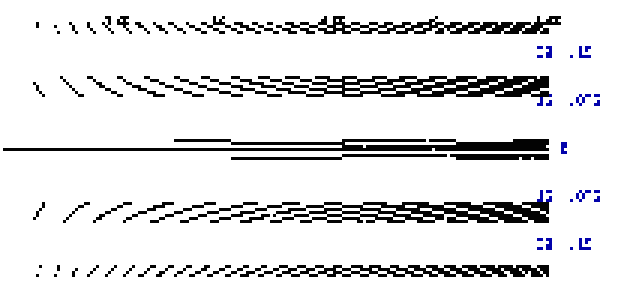Here is the computational portion of my program code for the original voting algorithm in QuickBASIC for DOS: TT = T TPER = INT(TT * PER / 100) TINC = INT(TPER / 1000) FOR VOTE = 1 TO TRI RANDOMIZE TIMER LOCATE 12, 5: PRINT "Number of trials :"; VOTE 50 B = 0: G = 0: C = 0: X = 0 INCRE = 0: ACT = 0: VARIA = 0 BP = TT / 2: GP = TT / 2 FOR C = 1 TO TPER V1 = RND(1) IF V1 < .5 THEN B = B + 1 IF V1 >= .5 THEN G = G + 1 ACT = ACT + 1 A = ACT / 2 100 INCRE = INCRE + 1 IF INCRE = TINC THEN INCRE = 0 COLOR 10 VARIA = INT((A - G) * (TT / ACT)) X = X + 1 PSET (X, VARIA / S) END IF 150 : NEXT C T = TT NEXT VOTE 200 I\$ = INKEY\$ IF I\$ = "" THEN 200 ========================================================= Here is my complete program code for the original voting algorithm in QuickBASIC for DOS: SCREEN 12 CLEAR CLS KEY(10) ON ON KEY(10) GOSUB 300 5 : VIEW (1, 1)-(639, 218) CLS KEY(9) ON ON KEY(9) GOSUB 400 COLOR 14 LOCATE 28, 5: PRINT "Exit - F10" COLOR 13 LOCATE 20, 46: PRINT "Current total ballots: "; TT LOCATE 22, 46: PRINT "Current scale: "; S LOCATE 24, 46: PRINT "Current percent: "; PER COLOR 11 LOCATE 20, 3: INPUT "Input total ballots"; T LOCATE 22, 3: INPUT "Scale (multiple of 1.5)"; S 6 LOCATE 24, 3: INPUT "Percent used for final projection"; PER IF PER = 10 OR PER = 20 OR PER = 40 OR PER = 80 OR PER = 99 THEN 7 LOCATE 22, 20: PRINT " ": GOTO 6 7 LOCATE 26, 3: INPUT "Number of trials"; TRI VIEW (1, 1)-(639, 479) CLS TT = T TPER = INT(TT * PER / 100) TINC = INT(TPER / 1000) YSCALE = TT / 750 VIEW (2, 218)-(600, 470), , 14 WINDOW (0, -YSCALE)-(1100, YSCALE) COL = 1 ROW = 7 COLOR 13 LOCATE 1, 1: PRINT "Start - press spacebar" LOCATE 2, 1: PRINT "Exit - F10 Restart - F9" COLOR 14 LOCATE 1, 34: PRINT "Total ballots: "; TT LOCATE 2, 34: PRINT "Scale: "; S LOCATE 3, 34: PRINT "Trials to run: "; TRI YLINE = INT(.9 * YSCALE) COLOR 8 LINE (100, YLINE)-(100, -YLINE) LINE (200, YLINE)-(200, -YLINE) LINE (300, YLINE)-(300, -YLINE) LINE (400, YLINE)-(400, -YLINE) LINE (500, YLINE)-(500, -YLINE) LINE (600, YLINE)-(600, -YLINE) LINE (700, YLINE)-(700, -YLINE) LINE (800, YLINE)-(800, -YLINE) LINE (900, YLINE)-(900, -YLINE) LINE (990, YLINE)-(990, -YLINE) L6 = INT(.75 * YSCALE) L5 = INT(.625 * YSCALE) L4 = INT(.5 * YSCALE) L3 = INT(.375 * YSCALE) L2 = INT(.25 * YSCALE) L1 = INT(.125 * YSCALE) COLOR 7 LINE (60, L6)-(990, L6) COLOR 8 LINE (60, L5)-(990, L5) LINE (60, L4)-(990, L4) COLOR 7 LINE (60, L3)-(990, L3) COLOR 8 LINE (60, L2)-(990, L2) LINE (60, L1)-(990, L1) COLOR 7 LINE (60, 0)-(990, 0) COLOR 8 LINE (60, -L1)-(990, -L1) LINE (60, -L2)-(990, -L2) COLOR 7 LINE (60, -L3)-(990, -L3) COLOR 8 LINE (60, -L4)-(990, -L4) LINE (60, -L5)-(990, -L5) COLOR 7 LINE (60, -L6)-(990, -L6) LOCATE 16, 67: PRINT INT(S * TT / 1000); (.1) * (S) LOCATE 19, 67: PRINT INT(S * TT / 2000); (.05) * (S) LOCATE 22, 67: PRINT " 0" LOCATE 25, 67: PRINT INT(S * TT / 2000); (.05) * (S) LOCATE 28, 67: PRINT INT(S * TT / 1000); (.1) * (S) COLOR 15 LOCATE 28, 14: PRINT "Variation of projection for A from actual A" COLOR 15 IF PER = 10 THEN 11 IF PER = 20 THEN 12 IF PER = 40 THEN 14 IF PER = 80 THEN 18 IF PER = 99 THEN 20 11 LOCATE 14, 14: PRINT "2%" LOCATE 14, 27: PRINT "4%" LOCATE 14, 41: PRINT "6%" LOCATE 14, 54: PRINT "8%" LOCATE 14, 68: PRINT "10%" GOTO 30 12 LOCATE 14, 14: PRINT "4%" LOCATE 14, 27: PRINT "8%" LOCATE 14, 41: PRINT "12%" LOCATE 14, 54: PRINT "16%" LOCATE 14, 68: PRINT "20%" GOTO 30 14 LOCATE 14, 14: PRINT "8%" LOCATE 14, 27: PRINT "16%" LOCATE 14, 41: PRINT "24%" LOCATE 14, 54: PRINT "32%" LOCATE 14, 68: PRINT "40%" GOTO 30 18 LOCATE 14, 14: PRINT "16%" LOCATE 14, 27: PRINT "32%" LOCATE 14, 41: PRINT "48%" LOCATE 14, 54: PRINT "64%" LOCATE 14, 68: PRINT "80%" GOTO 30 20 LOCATE 14, 14: PRINT "20%" LOCATE 14, 27: PRINT "40%" LOCATE 14, 41: PRINT "60%" LOCATE 14, 54: PRINT "80%" LOCATE 14, 68: PRINT "99%" 30 : COLOR 13 LOCATE 13, 2: PRINT "Press spacebar to continue" 40 I\$ = INKEY\$ IF I\$ = "" THEN 40 LOCATE 13, 2: PRINT " " FOR VOTE = 1 TO TRI RANDOMIZE TIMER LOCATE 12, 5: PRINT "Number of trials :"; VOTE 50 B = 0: G = 0: C = 0: X = 0 INCRE = 0: ACT = 0: VARIA = 0 BP = TT / 2: GP = TT / 2 FOR C = 1 TO TPER V1 = RND(1) IF V1 < .5 THEN B = B + 1 IF V1 >= .5 THEN G = G + 1 ACT = ACT + 1 A = ACT / 2 100 INCRE = INCRE + 1 IF INCRE = TINC THEN INCRE = 0 COLOR 10 VARIA = INT((A - G) * (TT / ACT)) X = X + 1 PSET (X, VARIA / S) END IF 150 : NEXT C T = TT NEXT VOTE 200 I\$ = INKEY\$ IF I\$ = "" THEN 200 COLOR 12 LINE (100, YLINE)-(100, -YLINE) LINE (200, YLINE)-(200, -YLINE) LINE (300, YLINE)-(300, -YLINE) LINE (400, YLINE)-(400, -YLINE) LINE (500, YLINE)-(500, -YLINE) LINE (600, YLINE)-(600, -YLINE) LINE (700, YLINE)-(700, -YLINE) LINE (800, YLINE)-(800, -YLINE) LINE (900, YLINE)-(900, -YLINE) LINE (990, YLINE)-(990, -YLINE) COLOR 12 LINE (60, L6)-(990, L6) COLOR 12 LINE (60, L5)-(990, L5) LINE (60, L4)-(990, L4) COLOR 12 LINE (60, L3)-(990, L3) COLOR 12 LINE (60, L2)-(990, L2) LINE (60, L1)-(990, L1) COLOR 12 LINE (60, 0)-(990, 0) COLOR 12 LINE (60, -L1)-(990, -L1) LINE (60, -L2)-(990, -L2) COLOR 12 LINE (60, -L3)-(990, -L3) COLOR 12 LINE (60, -L4)-(990, -L4) LINE (60, -L5)-(990, -L5) COLOR 12 LINE (60, -L6)-(990, -L6) 250 I\$ = INKEY\$ IF I\$ = "" THEN 250 GOTO 5 300 KEY(10) OFF: KEY(9) OFF END 400 KEY(9) OFF: GOTO 5 ================================================= Here is my original code for the VisualBASIC version. Private Sub voterunbtn_Click() Set viewport.Picture = LoadPicture("") DONE\$ = "done" per = Val(perbox.Text) amp = Val(ampbox.Text) inc = Val(incbox.Text) iter = Val(iterbox.Text) trials = Val(trialsbox.Text) iterper = Int(iter * (per / 100)) For total = 1 To trials Randomize Timer X = 0: G = 0: A = 0 For C = 1 To iterper RN = Rnd(1) If RN >= 0.5 Then G = G + 1 A = A + 1 A2 = A / 2 Y = (A2 - G) * (iter / A) X = X + ((100 * inc) / per) viewport.PSet (X, Y / amp), RGB(0, 0, 0) Next C Next total finalnum.Text = DONE\$ End Sub ================================================= home Balloting Simple trig Logistic map The Attractor of Henon Number doubling Barnsley's Fern The Sierpinski Triangle ```